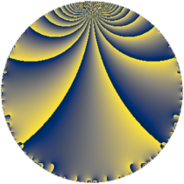# Properties

 Label 30.3.dLevel $30$ Weight $3$ Character orbit 30.d Rep. character $\chi_{30}(11,\cdot)$ Character field $\Q$ Dimension $4$ Newform subspaces $1$ Sturm bound $18$ Trace bound $0$

# Related objects

## Defining parameters

 Level: $$N$$ $$=$$ $$30 = 2 \cdot 3 \cdot 5$$ Weight: $$k$$ $$=$$ $$3$$ Character orbit: $$[\chi]$$ $$=$$ 30.d (of order $$2$$ and degree $$1$$) Character conductor: $$\operatorname{cond}(\chi)$$ $$=$$ $$3$$ Character field: $$\Q$$ Newform subspaces: $$1$$ Sturm bound: $$18$$ Trace bound: $$0$$

## Dimensions

The following table gives the dimensions of various subspaces of $$M_{3}(30, [\chi])$$.

Total New Old
Modular forms 16 4 12
Cusp forms 8 4 4
Eisenstein series 8 0 8

## Trace form

 $$4 q + 4 q^{3} - 8 q^{4} - 4 q^{6} + 8 q^{7} - 8 q^{9} + O(q^{10})$$ $$4 q + 4 q^{3} - 8 q^{4} - 4 q^{6} + 8 q^{7} - 8 q^{9} - 8 q^{12} - 40 q^{13} + 20 q^{15} + 16 q^{16} + 32 q^{18} + 32 q^{19} - 52 q^{21} + 48 q^{22} + 8 q^{24} - 20 q^{25} + 28 q^{27} - 16 q^{28} - 20 q^{30} + 32 q^{31} + 24 q^{33} - 96 q^{34} + 16 q^{36} - 88 q^{37} - 40 q^{39} - 128 q^{42} + 56 q^{43} + 20 q^{45} - 24 q^{46} + 16 q^{48} + 180 q^{49} + 72 q^{51} + 80 q^{52} + 140 q^{54} + 152 q^{57} - 40 q^{60} - 64 q^{61} - 256 q^{63} - 32 q^{64} + 48 q^{66} - 328 q^{67} - 132 q^{69} + 120 q^{70} - 64 q^{72} + 200 q^{73} - 20 q^{75} - 64 q^{76} + 40 q^{78} - 112 q^{79} + 28 q^{81} + 192 q^{82} + 104 q^{84} - 120 q^{85} + 240 q^{87} - 96 q^{88} - 80 q^{90} - 80 q^{91} + 32 q^{93} - 120 q^{94} - 16 q^{96} + 296 q^{97} - 192 q^{99} + O(q^{100})$$

## Decomposition of $$S_{3}^{\mathrm{new}}(30, [\chi])$$ into newform subspaces

Label Dim $A$ Field CM Traces $q$-expansion
$a_{2}$ $a_{3}$ $a_{5}$ $a_{7}$
30.3.d.a $4$ $0.817$ $$\Q(\sqrt{-2}, \sqrt{-5})$$ None $$0$$ $$4$$ $$0$$ $$8$$ $$q-\beta _{2}q^{2}+(1+\beta _{1}-\beta _{2}+\beta _{3})q^{3}-2q^{4}+\cdots$$

## Decomposition of $$S_{3}^{\mathrm{old}}(30, [\chi])$$ into lower level spaces

$$S_{3}^{\mathrm{old}}(30, [\chi]) \cong$$ $$S_{3}^{\mathrm{new}}(15, [\chi])$$$$^{\oplus 2}$$